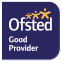﻿ Mathematics - St Birinus School
Search Twitter# Mathematics

#### KS3 Mathematics

KS3 Mathematics

KS3 Mathematics

Intent

The maths KS3 curriculum is based on a Mastery model which means taking the time to go deeper into student’s understanding using variation and mathematical reasoning. Students develop their mathematical fluency and thorough understanding without resorting to rote learning and are confidently able to solve non-routine maths problems. The Mastery approach is completely inclusive for all learners which means that all students are confident and prepared. Students are rigorously challenged in this, the first part of their five-year journey of secondary school mathematics. Their fluency and understanding is checked regularly using feedback sessions within a lesson, ensuring all students are assessing their mathematical knowledge.

Curriculum sequence

 Term 1 Term 2 Term 3 Term 4 Term 5 Term 6 Year 7 Arithmetic laws; Negative numbers Negative numbers; Algebra skills Order of operations; Solving equations Fractions Ratio and proportion Angles Year 8 Types of Number; Solving Equations Straight Line Graphs Angles; Shape properties Ratio; Proportional reasoning Fractions Statistics

Developing Skills

• accurately recall facts, maths specific vocabulary and definitions
• use and interpret notation correctly
• accurately carry out methods or tasks requiring multi-step solutions
• persuade others using mathematical reasoning
• interpret and communicate information accurately
• critically evaluate a given piece of maths, including the methods used and results obtained
• translate problems, including in context, into a series of mathematical processes
• make and use connections between different parts of mathematics
• interpret results in the context of the given problem and evaluate these solutions to identify any possible assumptions

The maths curriculum at St Birinus has been mapped against the framework of the national curriculum and in the five-year curriculum journey in maths we meet its breadth and ambition as we roll out the Mastery model through the year groups with the ultimate aim of a seven-year curriculum culminating in A level maths for Year 13.

Meeting the needs of SEND and disadvantaged students

In line with whole-school priorities, maths teachers place SEND and disadvantaged students at the centre of our lesson planning.  Key to ensuring this is high quality teaching and learning alongside routine, embedded feedback which enables students to regularly check their understanding and recall of knowledge and procedures. We ensure disadvantaged students are included in hands down questioning, check their understanding during lessons and have well established routines for clear and regular communication with parents and carers.

Good practice for SEND and disadvantaged students benefits all students at St Birinus and these strategies are particularly supportive for vulnerable learners:

• Taking a central role in mathematical reasoning and discussion
• Routines are clear and consistent across the department. With consistent basic knowledge starters in both Year 7 and 8
• Feedback sessions are conducted every six lessons, giving students the opportunity to assess their confidence and understanding of mathematical procedures and further examples and practice to embed concepts and methods
• Tracking systems are in place to monitor student performance throughout the course of the year so that we can be sure of the progress made and early interventions are in place where necessary

Retention

The depth and reasoning implicit in the mastery model means students are fully grasping concepts and not rushing to move on to the next ‘isolated’ topic.

Homework

In Key stage 3 maths students are set 30 minutes of homework a week to establish good study habits. Homework tasks are either focused on embedding new subject or specific revision tasks for end of topic assessments.

Assessment

Students are assessed regularly during topics in feedback session and three times more formally throughout the course of the academic year at the end of each long term.

Feedback sessions give students the opportunity to retrieve and apply key knowledge from the module they have been learning and the more formal end of term assessments are designed to let students be successful both in method and problem-solving tasks, giving students some experience of summative testing. The tests include a ‘knowledge check’ section, designed to assess their ability to recall and apply mathematical methods, alongside a ‘problem solving’ section which gives them some experience of the more contextual applied questions. A feedback session then identifies any areas in which they need to develop.

Mrs Gothard, Faculty Leader for Mathematics

zgothard@st-birinus.oxon.sch.uk

#### GCSE Mathematics

GCSE Mathematics

Programme of study

There are two tiers of study for maths in KS4, foundation and higher tiers. Foundation tier includes grades 1 to 5 and the higher tier includes grades 4 to 9 (grade 3 allowed).

Both tiers will provide students with the skills to develop fluent knowledge and understanding of mathematical methods and concepts, and to reason mathematically allowing them to comprehend and interpret mathematical information; empowering every student.

Course Content

Both tiers will cover the six main strands of mathematics:

Number

Algebra

Ratio and Proportion

Geometry and Measures

Probability

Statistics

Including the following:

Number structure and calculation

Fractions, decimals and percentages

Measures and accuracy

Algebraic notation, vocabulary and manipulation

Graphs

Solving equations and inequalities

Sequences

Ratio, proportion and rates of change

Geometry and measures properties and constructions

Mensuration and calculation

Vectors

Probability

Statistics

Assessment

Examination Board:

EDEXCEL

1 – 9 (9 is highest)

Assessment:

100% Exam

3 Examination Papers, each for 1 hour 30 minutes

Each paper has a range of question types, from straight forward method questions to problem solving in context situations.

Paper 1 – Non-Calculator 80 Marks

Paper 2 – Calculator 80 Marks

Paper 3 – Calculator 80 Marks

Progression to Post-16:

A-Level Mathematics.

Contact